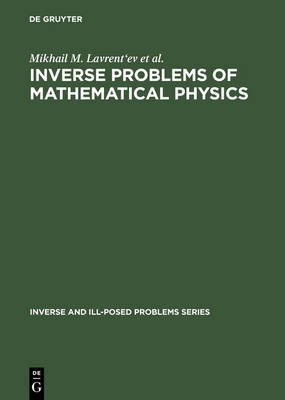•# Inverse Problems of Mathematical Physics - Inverse and Ill-Posed Problems Series 44 (Hardback)

(author), (author), (author)
£199.00
Hardback 275 Pages / Published: 27/09/2003
• Not available

This product is currently unavailable

This product is currently unavailable.

01/07 This title is now available from Walter de Gruyter. Please see www.degruyter.com for more information. The theory of Inverse Problems of Mathematical Physics is a vast and intensively developing field of modern mathematics. This book deals mostly with the theoretical aspects and treats some applications, as well as numerical methods of solving problems. In the introductory chapter the concept of mathematical modelling is given, the general differences between direct and inverse problems are outlined and strict mathematical definitions of correct and ill-posed problems are given. The following chapters deal with: - Approximate methods of solution of ill-posed problems - Integral geometry problems - One dimensional inverse problems - Inverse problems for the coupled Maxwell and Lame systems - Numerical solution of inverse problems: some examples This books is intended to serve as an introduction for specialists in other fields, and thus emphasizes the basic general principles and approaches to the solution of various problems, whereby concrete examples of results obtained are given.

Publisher: Brill
ISBN: 9789067643962
Number of pages: 275
Weight: 641 g
Dimensions: 244 x 170 x 18 mm